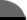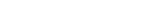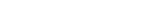Facts By Category:» Physics  » Astronomy  » Chemistry  » Biology  » Mathematics  » Geology  » Engineering  » Medicine  » Science  ScienceIQ Team:»Writers & EditorsxUmp.com
Science Supplies,
Toys & Gifts

PhysLink.com
Physics & Astronomy
Online
What Is The Pythagorean Theorem?Pythagoras was a famous Greek mathematician. He was particularly interested in the properties of triangles, and discovered a simple, fundamental relationship between the lengths of the sides of right triangles. The theory that he put forward from this relationship became fundamental to the practice of geometry (from the Greek words egeosi and emetrosi, meaning 'earth' and emeasurei and together meaning eearth measuring). To this day, the Pythagorean Theorem is used in geometry and algebra lessons. The theory of Pythagoras states that the square of the hypotenuse of a right triangle is equal to the sum of the squares of the other two sides. Pythagoras observed that if one made a square of each of the sides of a right triangle, then the area of the square on the longest side (the hypotenuse) was the same as the areas of the squares on the other two sides added together.

If the length of the hypotenuse is called A, and the lengths of the other two sides are called B and C, then A2 = B2 + C2. By using accurate measurement of distances and angles, and applying Pythagorasis theorem, it is possible to easily determine distances that are otherwise difficult to measure accurately. The process of measuring things in this way is called triangulation. It is very easy to convince yourself that the theory is true: just draw some right triangles and do the calculations. For example, using graph paper and a ruler, one can easily draw a triangle whose right-angled sides are three units and four units long (the units donit matter and can be centimeters, inches, feet, yards, meters, anything). The hypotenuse that joins the ends of these two lines will be five units in length.

Using this method it is possible to accurately draw lines whose lengths are difficult to measure. This is a commonly-used trick for indeterminate numbers such as the square roots of 2, 3, 5, 7, and others.

482

About the AuthorRichard M. J. Renneboog, MS Richard M. J. Renneboog is an independent private technical consultant and writer in both chemical and computer applications. Endeavors have included preparation of scripts for instructional and promotional video, corporate website design, curriculum development for training in advanced composites technology, and development.

Further ReadingPythagorean Triangle: or The Science of Numbersby George Oliver

Related Web Links
 Pythagorean Theorem by Alexander Bognmolny Animated Proof of the Pythagorean Theorem by USNA.edu

 Home | Privacy Policy | Cookie PolicyCopyright © 2002-2019 ScienceIQ.com - All Rights Reserved